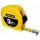# Salad

We need two tenths kg of a carrot, one tenth of peas and three tenths of of tomatoes to make salad. Express the fraction of the weight of the vegetables to be salad. Convert the result to grams.

Result

f =  0.6 kg
g =  600 g

#### Solution:Leave us a comment of example and its solution (i.e. if it is still somewhat unclear...):

Showing 0 comments:Be the first to comment!#### To solve this example are needed these knowledge from mathematics:

Need help calculate sum, simplify or multiply fractions? Try our fraction calculator. Do you want to convert mass units?

## Next similar examples:

1. RecipeA recipe requires 2 pounds of flour. If a chef wants to triple the recipe, how many ounces of flour will be needed?
2. Fraction and a decimalWrite as a fraction and a decimal. One and two plus three and five hundredths
3. Pound2kilosHow many pounds make 1 kilograms?
4. In fractionsAn ant climbs 2/5 of the pole on the first hour and climbs 1/4 of the pole on the next hour. What part of the pole does the ant climb in two hours?
5. ZdeněkZdeněk picked up 15 l of water from a 100-liter full-water barrel. Write a fraction of what part of Zdeněk's water he picked.
6. Fraction to decimalWrite the fraction 3/22 as a decimal.
7. Lengths of the poolMiguel swam 6 lengths of the pool. Mat swam 3 times as far as Miguel. Lionel swam 1/3 as far as Miguel. How many lengths did mat swim?
8. Mixed2improperWrite the mixed number as an improper fraction. 166 2/3
9. Adding mixed numbersAdd this two mixed numbers: 1 5/6 + 2 2/11=
10. NotebooksLiza a store owner buys 560 notebooks. He sold 3/8 of the notebook then she adds the stocks of notebook of 1/4 of the number of notebooks she bought. What is the total number of notebook she bought?
11. 3 children3 children had to divide 4 pounds is candy. How much candy did each child get?
12. Roses and tulipsAt the florist are 50 tulips and 5 times less roses. How many flowers are in flower shop?
13. Adding mixed numerals3 3/4 + 2 3/5 + 5 1/2 Show your solution.
14. Brick weightHalf the weight of a brick plus 20 pounds is equal to 1/3 the weight of the brick plus 30 pounds. How much does the brick weigh?
15. Addition of mixed numeralsAdd two mixed fractions: 2 4/6 + 1 3/6
16. Sum of fractionsWhat is the sum of 2/3+3/5?
17. Conversion of unitsComplete the following length data